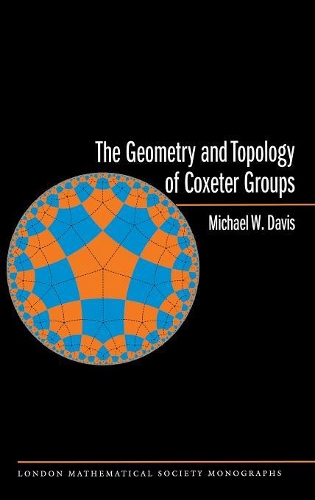•# The Geometry and Topology of Coxeter Groups. (LMS-32) - London Mathematical Society Monographs (Hardback)

(author)
£104.00
Hardback 600 Pages / Published: 30/11/2007
• We can order this

Usually dispatched within 3 weeks

The Geometry and Topology of Coxeter Groups is a comprehensive and authoritative treatment of Coxeter groups from the viewpoint of geometric group theory. Groups generated by reflections are ubiquitous in mathematics, and there are classical examples of reflection groups in spherical, Euclidean, and hyperbolic geometry. Any Coxeter group can be realized as a group generated by reflection on a certain contractible cell complex, and this complex is the principal subject of this book. The book explains a theorem of Moussong that demonstrates that a polyhedral metric on this cell complex is nonpositively curved, meaning that Coxeter groups are "CAT(0) groups." The book describes the reflection group trick, one of the most potent sources of examples of aspherical manifolds. And the book discusses many important topics in geometric group theory and topology, including Hopf's theory of ends; contractible manifolds and homology spheres; the Poincare Conjecture; and Gromov's theory of CAT(0) spaces and groups. Finally, the book examines connections between Coxeter groups and some of topology's most famous open problems concerning aspherical manifolds, such as the Euler Characteristic Conjecture and the Borel and Singer conjectures.

Publisher: Princeton University Press
ISBN: 9780691131382
Number of pages: 600
Weight: 992 g
Dimensions: 235 x 152 x 33 mm